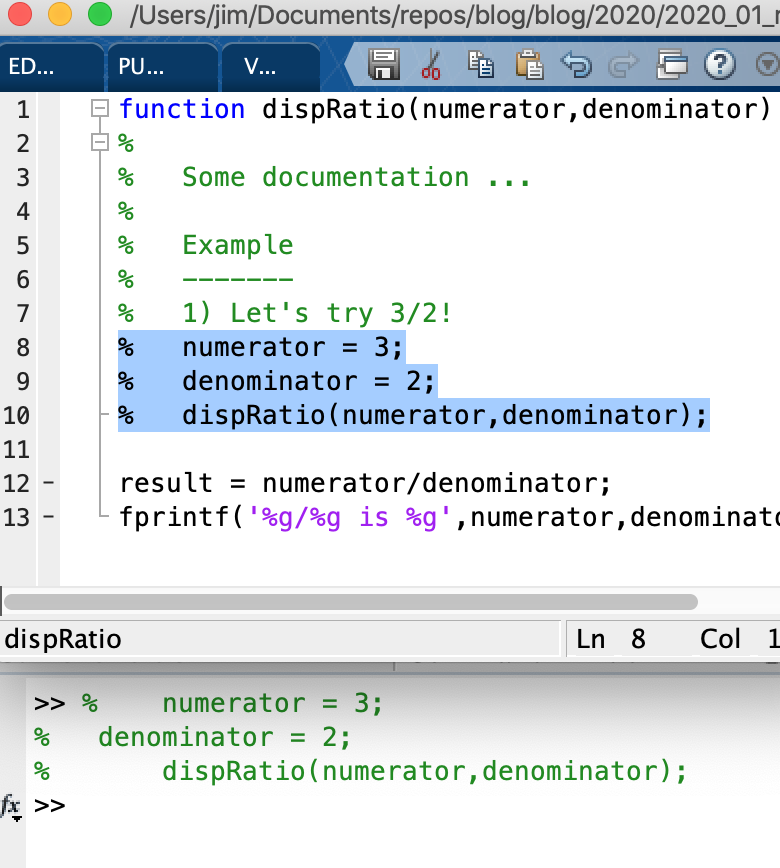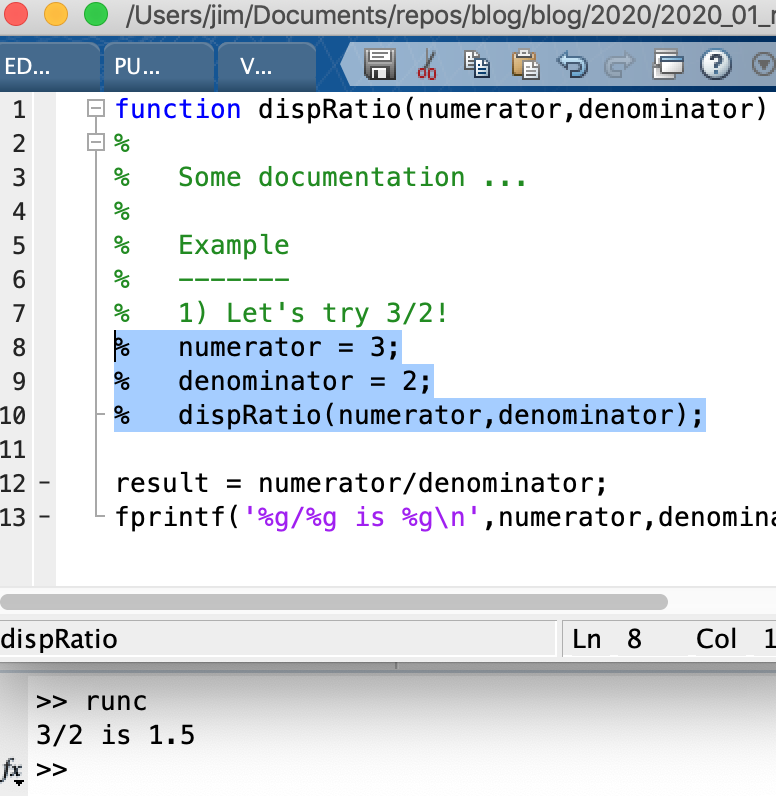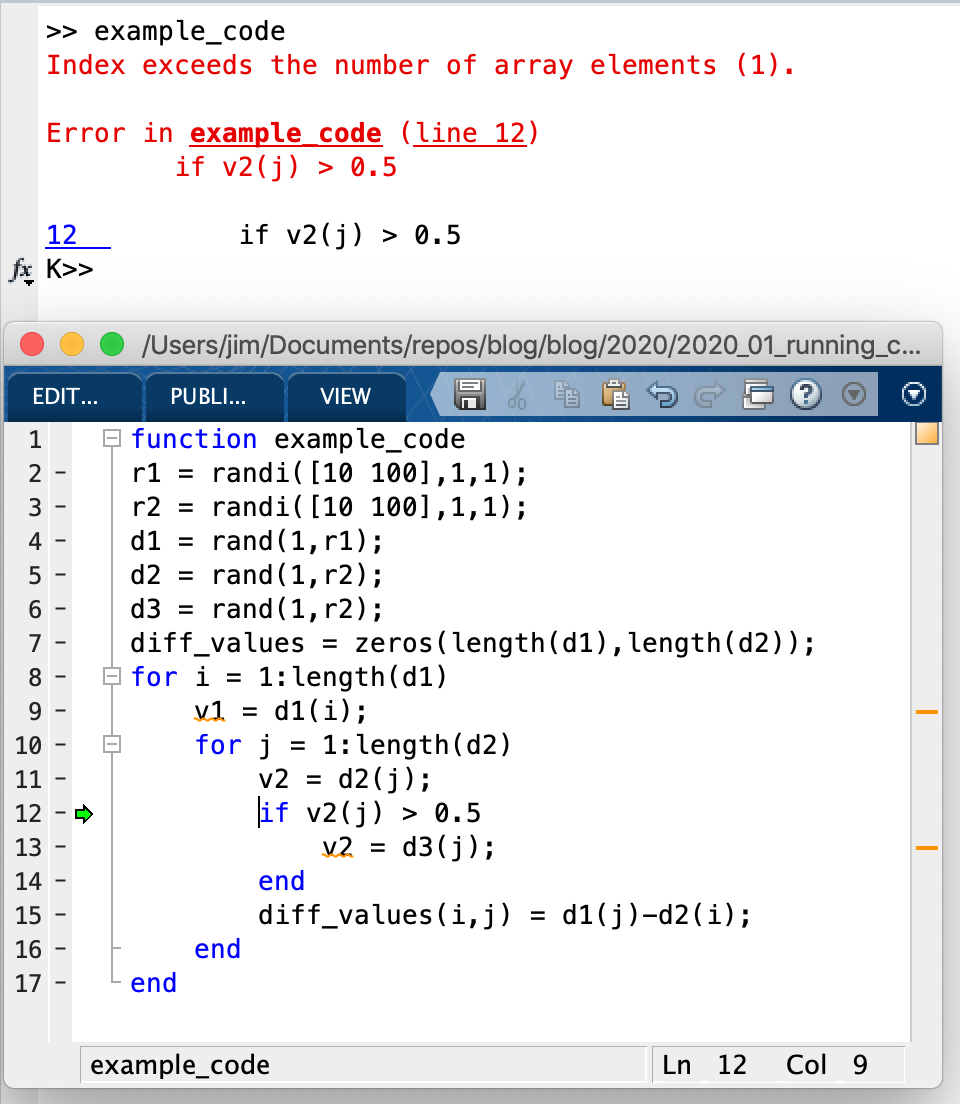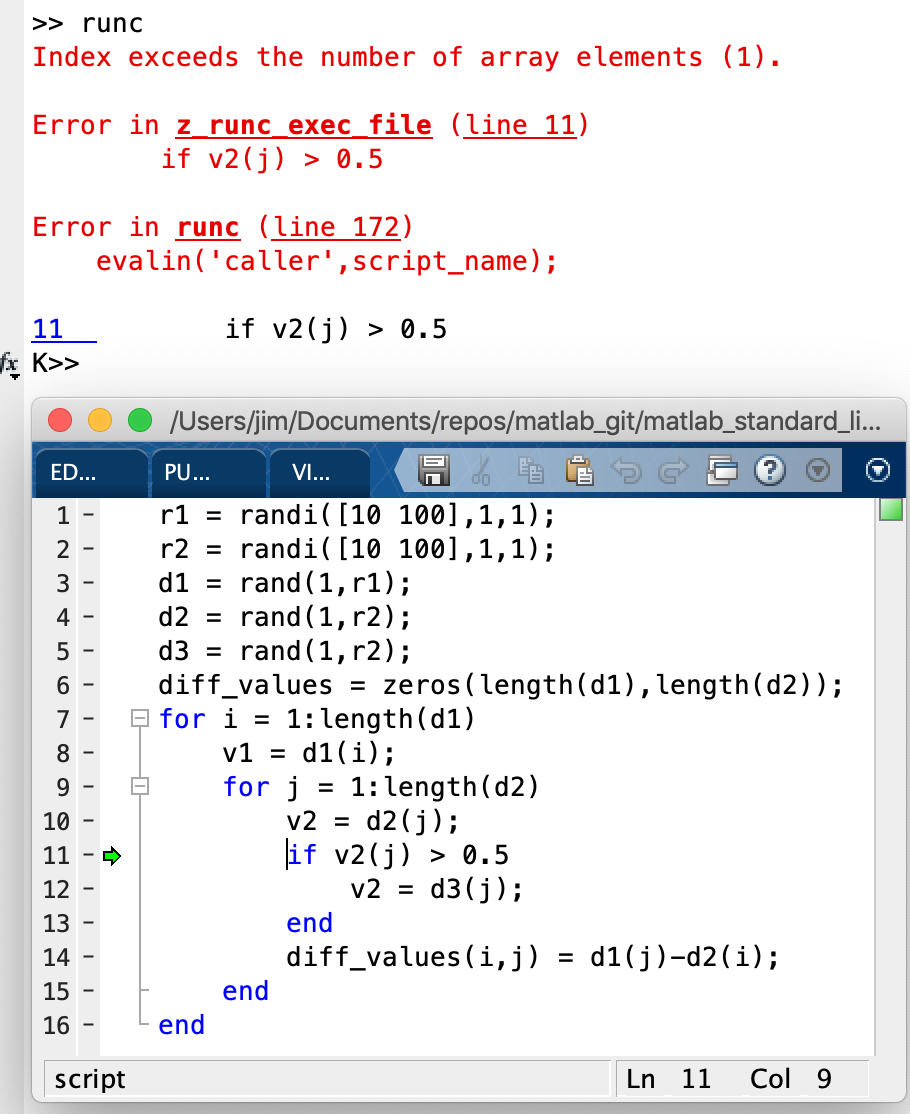# Running clipboard code Matlab

This post will discuss a Matlab function, runc I created. The function helps with 1) running commented examples and 2) with debugging highlighted/selected then evaluated code blocks.

The code is kept my “Matlab Standard Library” repo. The library was started in June 2013 as a way of organizing reusable Matlab functions and classes that I write. The code library is a topic for another post. In the mean time it is just useful to know that runc is located in this library link

## Running example code

Initially runc was written to run example code in documentation comments. This isn’t necessary for functions when using Matlab’s help function since help removes all comment characters. However, when inspecting code in the editor the comments remain and evaluating highlighted code with comments won’t run the code. For example:The example above has been highlighted and evaluated (right-click => evaluate selection). Not surprisingly the command window only displays the comments, it doesn't run the code.

Now, if instead of evaluating the selection, we copy it to the clipboard, we can use runc at the command window to evaluate the commented code:Now we've copied the selection rather than evaluating it. By typing runc in the command window we actually evaluate the code in the comments, rather than just displaying the commented code.

An alternative approach to running commented examples is to uncomment multiple lines of code, evaluate the selection, then undo the uncommenting. This approach works but I don’t like making changes to the file. In particular, it is fairly easy to forget to recomment the code and to save the file. This leads to infinite recursion!

Another approach for examples is to use multi-line comments (by using %{ and %} character pairs) . For example:

function dispRatio()
%
%	some documentation ...
%{
%Start of comment

%Example
%-------
%1) Let's try 3/2!
numerator = 3;
denominator = 2;
dispRatio(numerator,denominator);

%End of multiline comment
%}

result = numerator/denominator;
fprintf('%g/%g is %g\n',numerator,denominator,result)

end


In the above example, the example in the multi-line comment can evaluated directly, since the code lines don’t contain the comment character. However, Matlab doesn’t support multi-line comments for documentation, so typing help dispRatio will not show the example! Thus multi-line comments are really only useful for internal examples/testing.

So in summary one function of runc is to allow evaluation of commented lines that have been copied to the clipboard.

## Better debugging

It turns out that the way runc works also makes it useful for debugging evaluated code, whether that code is commented out or not. More specifically, using runc provides additional debug information that is not available when simply evaluating selected lines.

Consider the following code:

r1 = randi([10 100],1,1);
r2 = randi([10 100],1,1);
d1 = rand(1,r1);
d2 = rand(1,r2);
d3 = rand(1,r2);
diff_values = zeros(length(d1),length(d2));
for i = 1:length(d1)
v1 = d1(i);
for j = 1:length(d2)
v2 = d2(j);
if v2(j) > 0.5
v2 = d3(j);
end
diff_values(i,j) = d1(j)-d2(i);
end
end


The specifics of the above code are not critical. What is important is that the code has an error in it. Selecting and evaluating this code throws the following error:

Index exceeds the number of array elements (1).


If we ask Matlab for more details, we get the following:

>> lasterror

ans =

struct with fields:

message: 'Index exceeds the number of array elements (1).'
stack: [0×1 struct]


In the above you should notice that the stack trace is empty. Basically, Matlab won’t tell us where the error occurred!

In addition to not telling us where the error occurred, it doesn’t stop execution at the error so that we can debug. Debugging on error is not enabled by default, but can be enabled with the command dbstop if error. I personally place this command in my startup script. This can slow code slightly - or used to, I haven’t tried it in a while - but basically this command states that the Matlab interpreter should enter debug mode when an error in execution occurs. This allows you to evaluate variables in the context of the error, rather than trying to manually set a debug point to inspect the error.

If our code were executed as a function or script, rather than by evaluating, we would stop at the line of our error:The debugger has stopped at the line of the error (see green arrow) and is ready for debugging in the command window as indicated by 'K>>'. This occurs because we ran our code as its own function, rather than evaluating selected lines.

Importantly, the part you are evaluating might not be the entirety of a function or script, but rather a part of one. Thus our goal is to somehow get debugging at the point of an error when evaluating highlighted code. runc allows for this.

If we copy the highlighted lines, then type runc, we get the debugging we are looking for:Figure: Like the above image, we are stopped in the debugger. If you look at the printed stack trace the error didn't come from the file we highlighted, but rather from a file called z_runc_exec_file. How this works is discussed in the next section. Importantly though, we get debugging from highlighting and "executing" the selected/copied code.

One tricky usage note: the code actually gets run in a temporary file that can look very much like the original file. Don’t make edits to the temporary file!

## Execution Details

runc works by grabbing the text that has been copied to the clipboard and writing it to a specific file z_runc_exec_file that is on the path. This file (script) is then executed by calling evalin('caller',script_name);. Since we are using a script (and not a function) and since we evaluate the script in the caller, all the variables in the caller are available for running the code (either the workspace currently being debugged or the base workspace). In other words, this setup means we don’t need to worry about passing in variables to the script or defining variables in the script, as long as they are defined in the caller. If code would work by highlighting and evaluating, then it will also work when written to a script and evaluated in runc’s caller.

The original runc code was meant for running commented code. When I wanted to expand the function to handle copied code that wasn’t commented (for better debugging), I had to figure out how to handle commented code in one case and uncommented (or semi-commented) code in another case. The approach I settled on was to determine whether there were any non-empty lines without comments. If the code finds any uncommented lines, it leaves any comments in place. If all lines have comments, then all lines are uncommented.

This code would have comments removed because all lines are commented.

% a = 1
% b = 2
% c = a + b


This code would keep comments because not all lines are commented.

% This code adds a & b!
a = 1
%a = 2 %old value
b = 2
c = a + b


## “Installing” the code

Installing using the standard library should be done as follows: 1) Placing the standard library base folder on your Matlab path. 2) Calling sl.initialize on startup (this is currently a bit heavier than I would like - downloads additional files …)

Alternatively, I’ve removed the dependencies and placed the single ‘runc’ file in the folder that holds this article: https://github.com/JimHokanson/blog/tree/master/blog/2020/2020_01_running_clipboard_code_matlab